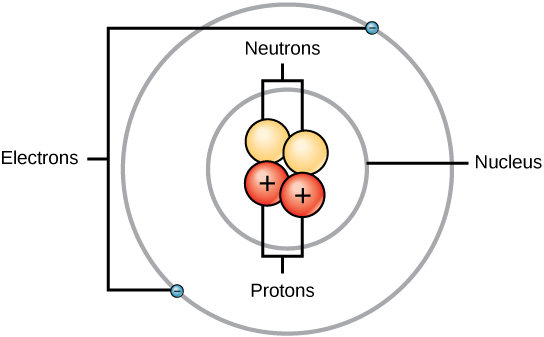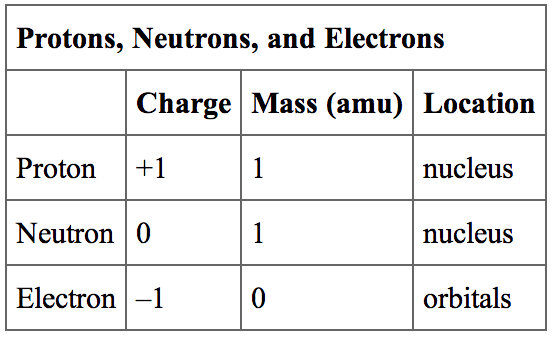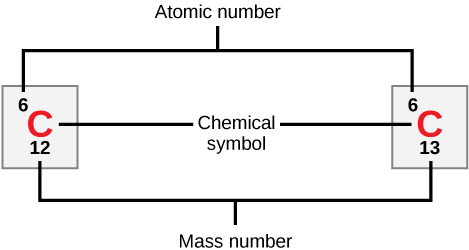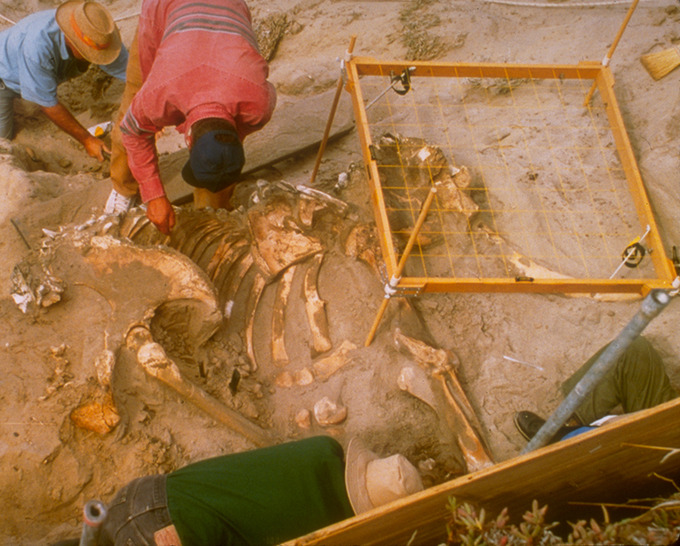## Overview of Atomic Structure

Atoms are made up of particles called protons, neutrons, and electrons, which are responsible for the mass and charge of atoms.

### Learning Objectives

Discuss the electronic and structural properties of an atom

### Key Takeaways

#### Key Points

• An atom is composed of two regions: the nucleus, which is in the center of the atom and contains protons and neutrons, and the outer region of the atom, which holds its electrons in orbit around the nucleus.
• Protons and neutrons have approximately the same mass, about 1.67 × 10-24 grams, which scientists define as one atomic mass unit (amu) or one Dalton.
• Each electron has a negative charge (-1) equal to the positive charge of a proton (+1).
• Neutrons are uncharged particles found within the nucleus.

#### Key Terms

• atom: The smallest possible amount of matter which still retains its identity as a chemical element, consisting of a nucleus surrounded by electrons.
• proton: Positively charged subatomic particle forming part of the nucleus of an atom and determining the atomic number of an element. It weighs 1 amu.
• neutron: A subatomic particle forming part of the nucleus of an atom. It has no charge. It is equal in mass to a proton or it weighs 1 amu.

An atom is the smallest unit of matter that retains all of the chemical properties of an element. Atoms combine to form molecules, which then interact to form solids, gases, or liquids. For example, water is composed of hydrogen and oxygen atoms that have combined to form water molecules. Many biological processes are devoted to breaking down molecules into their component atoms so they can be reassembled into a more useful molecule.

### Atomic Particles

Atoms consist of three basic particles: protons, electrons, and neutrons. The nucleus (center) of the atom contains the protons (positively charged) and the neutrons (no charge). The outermost regions of the atom are called electron shells and contain the electrons (negatively charged). Atoms have different properties based on the arrangement and number of their basic particles.

The hydrogen atom (H) contains only one proton, one electron, and no neutrons. This can be determined using the atomic number and the mass number of the element (see the concept on atomic numbers and mass numbers).Structure of an atom: Elements, such as helium, depicted here, are made up of atoms. Atoms are made up of protons and neutrons located within the nucleus, with electrons in orbitals surrounding the nucleus.

### Atomic Mass

Protons and neutrons have approximately the same mass, about 1.67 × 10-24 grams. Scientists define this amount of mass as one atomic mass unit (amu) or one Dalton. Although similar in mass, protons are positively charged, while neutrons have no charge. Therefore, the number of neutrons in an atom contributes significantly to its mass, but not to its charge.

Electrons are much smaller in mass than protons, weighing only 9.11 × 10-28 grams, or about 1/1800 of an atomic mass unit. Therefore, they do not contribute much to an element’s overall atomic mass. When considering atomic mass, it is customary to ignore the mass of any electrons and calculate the atom’s mass based on the number of protons and neutrons alone.

Electrons contribute greatly to the atom’s charge, as each electron has a negative charge equal to the positive charge of a proton. Scientists define these charges as “+1” and “-1. ” In an uncharged, neutral atom, the number of electrons orbiting the nucleus is equal to the number of protons inside the nucleus. In these atoms, the positive and negative charges cancel each other out, leading to an atom with no net charge.Protons, neutrons, and electrons: Both protons and neutrons have a mass of 1 amu and are found in the nucleus. However, protons have a charge of +1, and neutrons are uncharged. Electrons have a mass of approximately 0 amu, orbit the nucleus, and have a charge of -1.

Exploring Electron Properties: Compare the behavior of electrons to that of other charged particles to discover properties of electrons such as charge and mass.

### Volume of Atoms

Accounting for the sizes of protons, neutrons, and electrons, most of the volume of an atom—greater than 99 percent—is, in fact, empty space. Despite all this empty space, solid objects do not just pass through one another. The electrons that surround all atoms are negatively charged and cause atoms to repel one another, preventing atoms from occupying the same space. These intermolecular forces prevent you from falling through an object like your chair.

Interactive: Build an Atom: Build an atom out of protons, neutrons, and electrons, and see how the element, charge, and mass change. Then play a game to test your ideas!

## Atomic Number and Mass Number

The atomic number is the number of protons in an element, while the mass number is the number of protons plus the number of neutrons.

### Learning Objectives

Determine the relationship between the mass number of an atom, its atomic number, its atomic mass, and its number of subatomic particles

### Key Takeaways

#### Key Points

• Neutral atoms of each element contain an equal number of protons and electrons.
• The number of protons determines an element’s atomic number and is used to distinguish one element from another.
• The number of neutrons is variable, resulting in isotopes, which are different forms of the same atom that vary only in the number of neutrons they possess.
• Together, the number of protons and the number of neutrons determine an element’s mass number.
• Since an element’s isotopes have slightly different mass numbers, the atomic mass is calculated by obtaining the mean of the mass numbers for its isotopes.

#### Key Terms

• mass number: The sum of the number of protons and the number of neutrons in an atom.
• atomic number: The number of protons in an atom.
• atomic mass: The average mass of an atom, taking into account all its naturally occurring isotopes.

### Atomic Number

Neutral atoms of an element contain an equal number of protons and electrons. The number of protons determines an element’s atomic number (Z) and distinguishes one element from another. For example, carbon’s atomic number (Z) is 6 because it has 6 protons. The number of neutrons can vary to produce isotopes, which are atoms of the same element that have different numbers of neutrons. The number of electrons can also be different in atoms of the same element, thus producing ions (charged atoms). For instance, iron, Fe, can exist in its neutral state, or in the +2 and +3  ionic states.

### Mass Number

An element’s mass number (A) is the sum of the number of protons and the number of neutrons. The small contribution of mass from electrons is disregarded in calculating the mass number. This approximation of mass can be used to easily calculate how many neutrons an element has by simply subtracting the number of protons from the mass number. Protons and neutrons both weigh about one atomic mass unit or amu. Isotopes of the same element will have the same atomic number but different mass numbers.Atomic number, chemical symbol, and mass number: Carbon has an atomic number of six, and two stable isotopes with mass numbers of twelve and thirteen, respectively. Its average atomic mass is 12.11.

Scientists determine the atomic mass by calculating the mean of the mass numbers for its naturally-occurring isotopes. Often, the resulting number contains a decimal. For example, the atomic mass of chlorine (Cl) is 35.45 amu because chlorine is composed of several isotopes, some (the majority) with an atomic mass of 35 amu (17 protons and 18 neutrons) and some with an atomic mass of 37 amu (17 protons and 20 neutrons).

Given an atomic number (Z) and mass number (A), you can find the number of protons, neutrons, and electrons in a neutral atom. For example, a lithium atom (Z=3, A=7 amu) contains three protons (found from Z), three electrons (as the number of protons is equal to the number of electrons in an atom), and four neutrons (7 – 3 = 4).

## Isotopes

Isotopes are various forms of an element that have the same number of protons, but a different number of neutrons.

### Learning Objectives

Discuss the properties of isotopes and their use in radiometric dating

### Key Takeaways

#### Key Points

• Isotopes are atoms of the same element that contain an identical number of protons, but a different number of neutrons.
• Despite having different numbers of neutrons, isotopes of the same element have very similar physical properties.
• Some isotopes are unstable and will undergo radioactive decay to become other elements.
• The predictable half-life of different decaying isotopes allows scientists to date material based on its isotopic composition, such as with Carbon-14 dating.

#### Key Terms

• isotope: Any of two or more forms of an element where the atoms have the same number of protons, but a different number of neutrons within their nuclei.
• half-life: The time it takes for half of the original concentration of an isotope to decay back to its more stable form.
• radioactive isotopes: an atom with an unstable nucleus, characterized by excess energy available that undergoes radioactive decay and creates most commonly gamma rays, alpha or beta particles.
• radiocarbon dating: Determining the age of an object by comparing the ratio of the 14C concentration found in it to the amount of 14C in the atmosphere.

### What is an Isotope?

Isotopes are various forms of an element that have the same number of protons but a different number of neutrons. Some elements, such as carbon, potassium, and uranium, have multiple naturally-occurring isotopes. Isotopes are defined first by their element and then by the sum of the protons and neutrons present.

• Carbon-12 (or 12C) contains six protons, six neutrons, and six electrons; therefore, it has a mass number of 12 amu (six protons and six neutrons).
• Carbon-14 (or 14C) contains six protons, eight neutrons, and six electrons; its atomic mass is 14 amu (six protons and eight neutrons).

While the mass of individual isotopes is different, their physical and chemical properties remain mostly unchanged.

Isotopes do differ in their stability. Carbon-12 (12C) is the most abundant of the carbon isotopes, accounting for 98.89% of carbon on Earth. Carbon-14 (14C) is unstable and only occurs in trace amounts. Unstable isotopes most commonly emit alpha particles (He2+) and electrons. Neutrons, protons, and positrons can also be emitted and electrons can be captured to attain a more stable atomic configuration (lower level of potential energy ) through a process called radioactive decay. The new atoms created may be in a high energy state and emit gamma rays which lowers the energy but alone does not change the atom into another isotope. These atoms are called radioactive isotopes or radioisotopes.